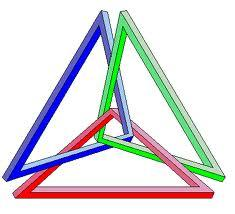# Isosceles right triangle

Calculate the area of an isosceles right triangle whose perimeter is 252 cm.

Correct result:

S =  2723.9 cm2

#### Solution:

Try calculation via our triangle calculator.We would be pleased if you find an error in the word problem, spelling mistakes, or inaccuracies and send it to us. Thank you!Tips to related online calculators
Pythagorean theorem is the base for the right triangle calculator.

#### You need to know the following knowledge to solve this word math problem:

We encourage you to watch this tutorial video on this math problem:

## Next similar math problems:

• Triangle SASCalculate the triangle area and perimeter, if the two sides are 51 cm and 110 cm long and angle them clamped is 130 °.
• Isosceles IIIThe base of the isosceles triangle is 17 cm area 416 cm2. Calculate the perimeter of this triangle.
• RhombusCalculate the perimeter and area of ​​a rhombus whose diagonals are 39 cm and 51 cm long.
• Right isoscelesCalculate area of the isosceles right triangle which perimeter is 41 cm.
• ISO trapezoid v2bases of Isosceles trapezoid measured 20 cm and 4 cm and its perimeter is 55 cm. What is the are of a trapezoid?
• Isosceles triangleCalculate area and perimeter of an isosceles triangle ABC with base AB if a = 6 cm, c = 7 cm.
• Square diagonalCalculate the length of the square diagonal if the perimeter is 172 cm.
• Isosceles trapezoidCalculate the area of an isosceles trapezoid whose bases are in the ratio of 4:3; leg b = 13 cm and height = 12 cm.
• Isosceles triangleCalculate the area of an isosceles triangle, the base of which measures 16 cm and the arms 10 cm.
• Similarity coefficientThe ratio of similarity of two equilateral triangles is 3.5 (ie 7:2). The length of the side of smaller triangle is 2.4 cm. Calculate the perimeter and area of ​​the larger triangle.
• DescribedCalculate perimeter of the circle described by a triangle with sides 478, 255, 352.
• Right isosceles triangleRight isosceles triangle has an altitude x drawn from the right angle to the hypotenuse dividing it into 2 equal segments. The length of one segment is 5 cm. What is the area of the triangle?
• Diamond diagonalsCalculate the diamonds' diagonals lengths if the diamond area is 156 cm square, and the side length is 13 cm.
• Rhombus IVCalculate the length of the diagonals of the rhombus, whose lengths are in the ratio 1: 2 and a rhombus side is 35 cm.
• Isosceles right triangleArea of an isosceles right triangle is 18 dm2. Calculate the length of its base.
• LogsThe trunk diameter is 52 cm. Is it possible to inscribe a square prism with side 36 cm?
• Axial sectionThe axial section of the cone is an equilateral triangle with area 168 cm2. Calculate the volume of the cone.